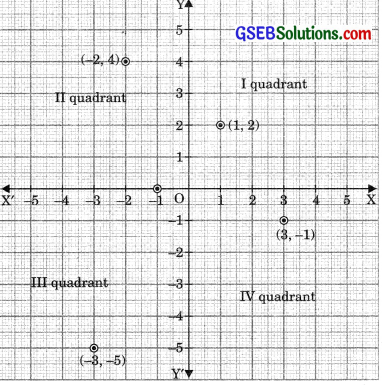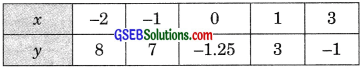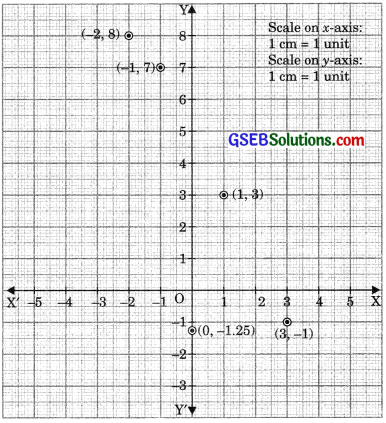# GSEB Solutions Class 9 Maths Chapter 3 Coordinate Geometry Ex 3.3

Gujarat Board GSEB Solutions Class 9 Maths Chapter 3 Coordinate Geometry Ex 3.3 Textbook Questions and Answers.

## Gujarat Board Textbook Solutions Class 9 Maths Chapter 3 Coordinate Geometry Ex 3.3

Question 1.
In which quadrant or on which axis do each of the points (-2, 4), (3, -1), (-1, 0), (1, 2) and (-3, -5) lie? verify your answer by locating them on the Cartesian plane.
Solution:
The point (-2, 4) lies in the II quadrant.
The point (3, -1) lies in the IV quadrant.
The point (-1, 0) lies on negative x-axis.
The point (1, 2) lies in the I quadrant.
The point (-3, -5) lies in the III quadrant.Question 2.
Plot the points (x,y) given in the following table on the plane choosing suitable units of distance on the axes.Solution: